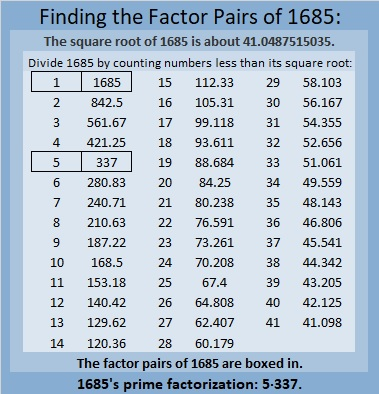1685 Oh, No! I’ve Created a Monster!

Contents

Today’s puzzle:

You may see some Frankenstein monsters walking about this time of year, but there’s no reason to be afraid of them or of this monster puzzle I’ve created. Simply write the numbers 1 to 12 in both factor areas so that the puzzle functions like a multiplication table.Here’s the same puzzle without any added color, if that’s what you prefer:Factors of 1685:

• 1685 is a composite number.
• Prime factorization: 1685 = 5 × 337.
• 1685 has no exponents greater than 1 in its prime factorization, so √1685 cannot be simplified.
• The exponents in the prime factorization are 1 and 1. Adding one to each exponent and multiplying we get (1 + 1)(1 + 1) = 2 × 2 = 4. Therefore 1685 has exactly 4 factors.
• The factors of 1685 are outlined with their factor pair partners in the graphic below.1685 is the sum of two squares in two different ways:
41² + 2² = 1685, and
34² + 23² = 1685.

1685 is the hypotenuse of FOUR Pythagorean triples:
164-1677-1685, calculated from 2(41)(2), 41² – 2², 41² + 2²,
627-1564-1685, calculated from 34² – 23², 2(34)(23), 34² + 23²,
875-1440-1685, which is 5 times (175-288-337), and
1011-1348-1685, which is (3-4-5) times 337.

This site uses Akismet to reduce spam. Learn how your comment data is processed.